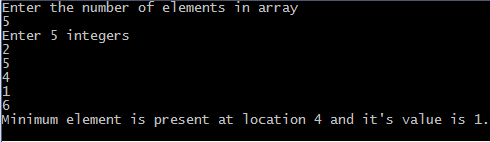## Print this Job Post# C program to find minimum element in array

## C programming code

#include <stdio.h>
#include<conio.h>
void main()
{
int array, minimum, size, c, location = 1;
printf("Enter the number of elements in array\n");
scanf("%d",&size);
printf("Enter %d integers\n", size);
for ( c = 0 ; c < size ; c++ )
scanf("%d", &array[c]);
minimum = array;
for ( c = 1 ; c < size ; c++ )
{
if ( array[c] < minimum )
{
minimum = array[c];
location = c+1;
}
}
printf("Minimum element is present at location %d and it's value is %d.\n", location, minimum);
getch();
}
Output  Of the Program:## C programming code using pointers

#include <stdio.h>
#include<conio.h>
void main()
{
int array, *minimum, size, c, location = 1;
printf("Enter the number of elements in array\n");
scanf("%d",&size);
printf("Enter %d integers\n", size);
for ( c = 0 ; c < size ; c++ )
scanf("%d", &array[c]);
minimum = array;
*minimum = *array;
for ( c = 1 ; c < size ; c++ )
{
if ( *(array+c) < *minimum )
{
*minimum = *(array+c);
location = c+1;
}
}
printf("Minimum element found at location %d and it's value is %d.\n", location, *minimum);
getch();
}